# ML | Logistic Regression using Tensorflow

• Difficulty Level : Hard
• Last Updated : 01 Nov, 2019

Prerequisites: Understanding Logistic Regression and TensorFlow.

Brief Summary of Logistic Regression:
Logistic Regression is Classification algorithm commonly used in Machine Learning. It allows categorizing data into discrete classes by learning the relationship from a given set of labeled data. It learns a linear relationship from the given dataset and then introduces a non-linearity in the form of the Sigmoid function.

In case of Logistic regression, the hypothesis is the Sigmoid of a straight line, i.e,whereWhere the vector w represents the Weights and the scalar b represents the Bias of the model.
Let us visualize the Sigmoid Function –

 import numpy as npimport matplotlib.pyplot as plt  def sigmoid(z):    return 1 / (1 + np.exp( - z))  plt.plot(np.arange(-5, 5, 0.1), sigmoid(np.arange(-5, 5, 0.1)))plt.title('Visualization of the Sigmoid Function')  plt.show()

Output: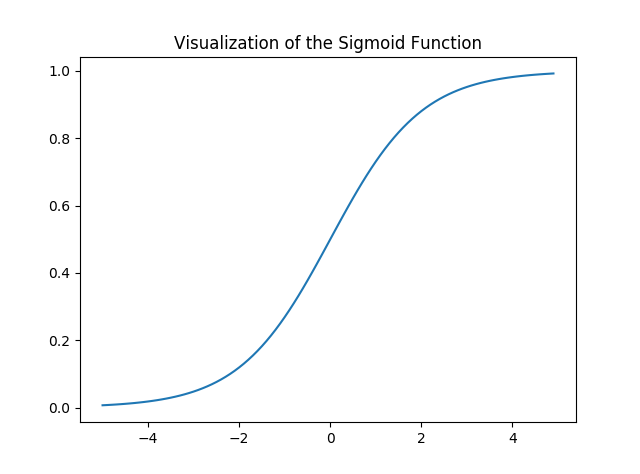Note that the range of the Sigmoid function is (0, 1) which means that the resultant values are in between 0 and 1. This property of Sigmoid function makes it a really good choice of Activation Function for Binary Classification. Also for z = 0, Sigmoid(z) = 0.5 which is the midpoint of the range of Sigmoid function.

Just like Linear Regression, we need to find the optimal values of w and b for which the cost function J is minimum. In this case, we will be using the Sigmoid Cross Entropy cost function which is given byThis cost function will then be optimized using Gradient Descent.

Implementation:
We will start by importing the necessary libraries. We will use Numpy along with Tensorflow for computations, Pandas for basic Data Analysis and Matplotlib for plotting. We will also be using the preprocessing module of Scikit-Learn for One Hot Encoding the data.

 # importing modulesimport numpy as npimport pandas as pdimport tensorflow as tfimport matplotlib.pyplot as pltfrom sklearn.preprocessing import OneHotEncoder

Next we will be importing the dataset. We will be using a subset of the famous Iris dataset.

 data = pd.read_csv('dataset.csv', header = None)print("Data Shape:", data.shape)  print(data.head())

Output:

Data Shape: (100, 4)
0    1    2  3
0  0  5.1  3.5  1
1  1  4.9  3.0  1
2  2  4.7  3.2  1
3  3  4.6  3.1  1
4  4  5.0  3.6  1

Now let’s get the feature matrix and the corresponding labels and visualize.

 # Feature Matrixx_orig = data.iloc[:, 1:-1].values  # Data labelsy_orig = data.iloc[:, -1:].values  print("Shape of Feature Matrix:", x_orig.shape)print("Shape Label Vector:", y_orig.shape)

Output:

Shape of Feature Matrix: (100, 2)
Shape Label Vector: (100, 1)

Visualize the given data.

 # Positive Data Pointsx_pos = np.array([x_orig[i] for i in range(len(x_orig))                                    if y_orig[i] == 1])  # Negative Data Pointsx_neg = np.array([x_orig[i] for i in range(len(x_orig))                                    if y_orig[i] == 0])  # Plotting the Positive Data Pointsplt.scatter(x_pos[:, 0], x_pos[:, 1], color = 'blue', label = 'Positive')  # Plotting the Negative Data Pointsplt.scatter(x_neg[:, 0], x_neg[:, 1], color = 'red', label = 'Negative')  plt.xlabel('Feature 1')plt.ylabel('Feature 2')plt.title('Plot of given data')plt.legend()  plt.show()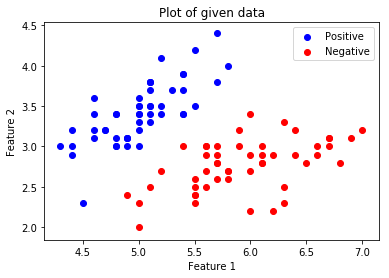.

Now we will be One Hot Encoding the data for it to work with the algorithm. One hot encoding transforms categorical features to a format that works better with classification and regression algorithms. We will also be setting the Learning Rate and the number of Epochs.

 # Creating the One Hot EncoderoneHot = OneHotEncoder()  # Encoding x_origoneHot.fit(x_orig)x = oneHot.transform(x_orig).toarray()  # Encoding y_origoneHot.fit(y_orig)y = oneHot.transform(y_orig).toarray()  alpha, epochs = 0.0035, 500m, n = x.shapeprint('m =', m)print('n =', n)print('Learning Rate =', alpha)print('Number of Epochs =', epochs)

Output:

m = 100
n = 7
Learning Rate = 0.0035
Number of Epochs = 500

Now we will start creating the model by defining the placeholders X and Y, so that we can feed our training examples x and y into the optimizer during the training process. We will also be creating the trainable Variables W and b which can be optimized by the Gradient Descent Optimizer.

 # There are n columns in the feature matrix# after One Hot Encoding.X = tf.placeholder(tf.float32, [None, n])  # Since this is a binary classification problem,# Y can take only 2 values.Y = tf.placeholder(tf.float32, [None, 2])  # Trainable Variable WeightsW = tf.Variable(tf.zeros([n, 2]))  # Trainable Variable Biasb = tf.Variable(tf.zeros())

Now declare the Hypothesis, Cost function, Optimizer and Global Variables Initializer.

 # HypothesisY_hat = tf.nn.sigmoid(tf.add(tf.matmul(X, W), b))  # Sigmoid Cross Entropy Cost Functioncost = tf.nn.sigmoid_cross_entropy_with_logits(                    logits = Y_hat, labels = Y)  # Gradient Descent Optimizeroptimizer = tf.train.GradientDescentOptimizer(         learning_rate = alpha).minimize(cost)  # Global Variables Initializerinit = tf.global_variables_initializer()

Begin the training process inside a Tensorflow Session.

 # Starting the Tensorflow Sessionwith tf.Session() as sess:          # Initializing the Variables    sess.run(init)          # Lists for storing the changing Cost and Accuracy in every Epoch    cost_history, accuracy_history = [], []          # Iterating through all the epochs    for epoch in range(epochs):        cost_per_epoch = 0                  # Running the Optimizer        sess.run(optimizer, feed_dict = {X : x, Y : y})                  # Calculating cost on current Epoch        c = sess.run(cost, feed_dict = {X : x, Y : y})                  # Calculating accuracy on current Epoch        correct_prediction = tf.equal(tf.argmax(Y_hat, 1),                                          tf.argmax(Y, 1))        accuracy = tf.reduce_mean(tf.cast(correct_prediction,                                                 tf.float32))                  # Storing Cost and Accuracy to the history        cost_history.append(sum(sum(c)))        accuracy_history.append(accuracy.eval({X : x, Y : y}) * 100)                  # Displaying result on current Epoch        if epoch % 100 == 0 and epoch != 0:            print("Epoch " + str(epoch) + " Cost: "                            + str(cost_history[-1]))          Weight = sess.run(W) # Optimized Weight    Bias = sess.run(b)   # Optimized Bias          # Final Accuracy    correct_prediction = tf.equal(tf.argmax(Y_hat, 1),                                      tf.argmax(Y, 1))    accuracy = tf.reduce_mean(tf.cast(correct_prediction,                                              tf.float32))    print("\nAccuracy:", accuracy_history[-1], "%")

Output:

Epoch 100 Cost: 125.700202942
Epoch 200 Cost: 120.647117615
Epoch 300 Cost: 118.151592255
Epoch 400 Cost: 116.549999237

Accuracy: 91.0000026226 %

Let’s plot the change of cost over the epochs.

 plt.plot(list(range(epochs)), cost_history)plt.xlabel('Epochs')plt.ylabel('Cost')plt.title('Decrease in Cost with Epochs')  plt.show()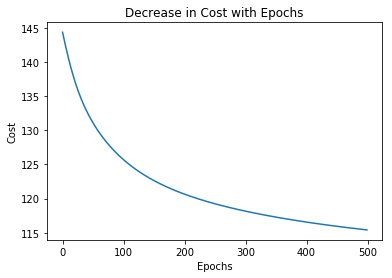Plot the change of accuracy over the epochs.

 plt.plot(list(range(epochs)), accuracy_history)plt.xlabel('Epochs')plt.ylabel('Accuracy')plt.title('Increase in Accuracy with Epochs')  plt.show()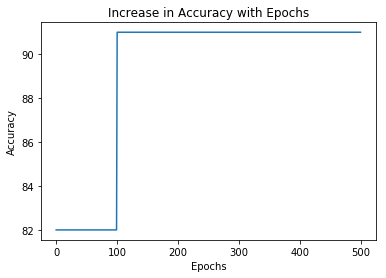Now we will be plotting the Decision Boundary for our trained classifier. A decision boundary is a hypersurface that partitions the underlying vector space into two sets, one for each class.

 # Calculating the Decision Boundarydecision_boundary_x = np.array([np.min(x_orig[:, 0]),                               np.max(x_orig[:, 0])])  decision_boundary_y = (- 1.0 / Weight) *      (decision_boundary_x * Weight + Bias)  decision_boundary_y = [sum(decision_boundary_y[:, 0]),                        sum(decision_boundary_y[:, 1])]  # Positive Data Pointsx_pos = np.array([x_orig[i] for i in range(len(x_orig))                                    if y_orig[i] == 1])  # Negative Data Pointsx_neg = np.array([x_orig[i] for i in range(len(x_orig))                                     if y_orig[i] == 0])  # Plotting the Positive Data Pointsplt.scatter(x_pos[:, 0], x_pos[:, 1],  color = 'blue', label = 'Positive')  # Plotting the Negative Data Pointsplt.scatter(x_neg[:, 0], x_neg[:, 1],   color = 'red', label = 'Negative')  # Plotting the Decision Boundaryplt.plot(decision_boundary_x, decision_boundary_y)plt.xlabel('Feature 1')plt.ylabel('Feature 2')plt.title('Plot of Decision Boundary')plt.legend()  plt.show()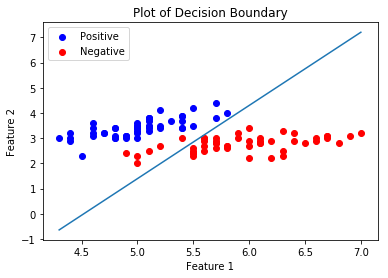My Personal Notes arrow_drop_up